# How to get the same or first day of next month on a given date in Excel?

This article briefs learners about the way to generate the same or the first day of the next month from the provided date in Excel. This article contains a stepwise explanation for both the discussed practices. The first example allows the learner to obtain the same-day date of the next month, this means if they pass “12-03-2023” as input then the generated output will be “12-05-2023”. Next example, allow leaner to get the first day of next month. This simply means if the input date “15-04-2023” then the generated output should be “01-05-2023”.

## Example 1: To get same day of next month from the provided date in Excel by using the formula:

### Step 1:

Consider the below given spreadsheet as a sample to understand the stepwise way to understand the processing steps taken to perform provided task. Please note that the date column contains entries with the format “month-date-year” format.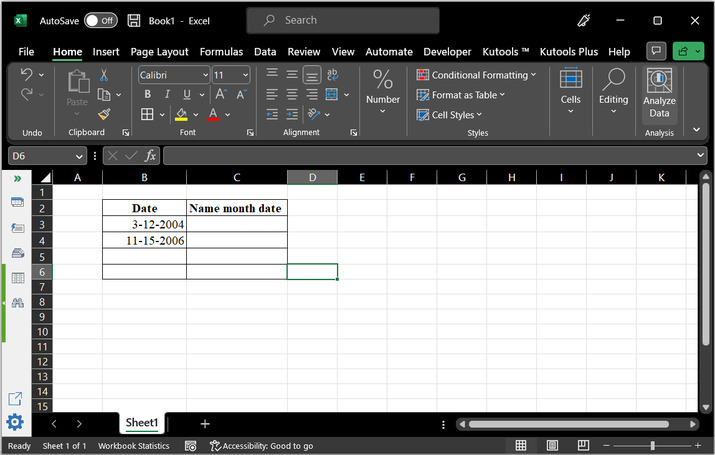### Step 2:

After that go to the column, where the learner is required to enter the next month's date. For this example, the taken cell is C3.

In the cell type “=EDATE(B3,1)” and press the “Enter” key.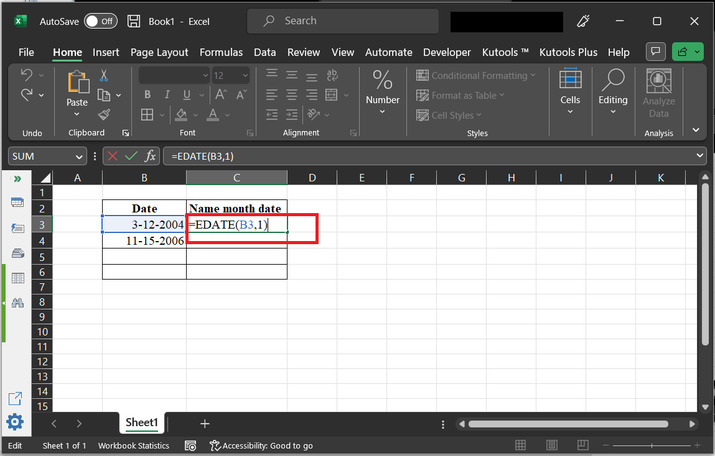### Explanation:

EDATE() function is used to calculate the future or past date by adding or subtracting a specified number of months from the provided date.

This function accept two parameters such as start date and month name.

Here, column B contains Start date.

And, 1, represent to add 1 month to the existing date. Please note if this value is positive then excel will return a future date, else the function will return the past date values.

### Step 3:

Press “Enter” key and drag the plus sign of the C3 cell to C4 cell, this will copy same formula for both the cells.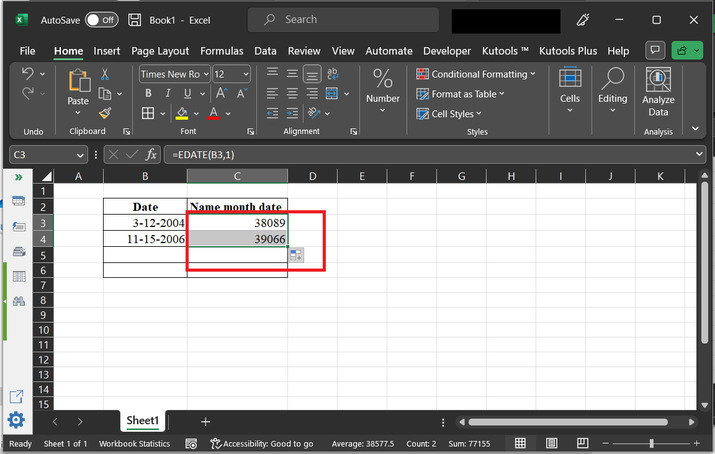### Step 4:

After that go to the C column, and select the whole column, by clicking on the cell header “C”. this will change the appearance of cell as provided below: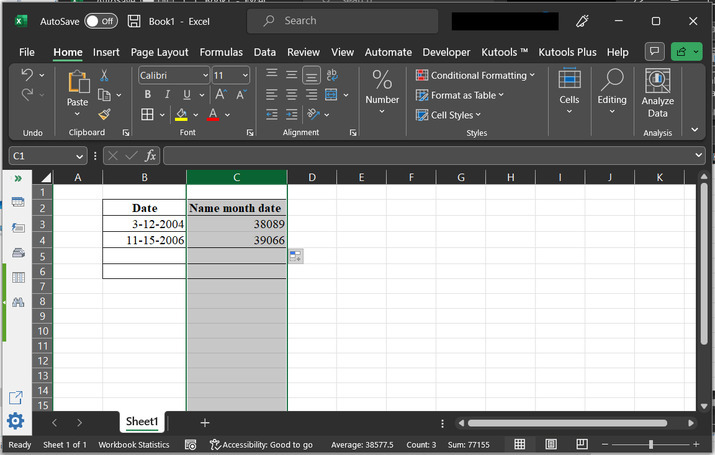### Step 5:

The next step includes choosing the “Number” from the style section, and then clicking on the appeared drop-down arrow. Finally, select the option of “Short Date”.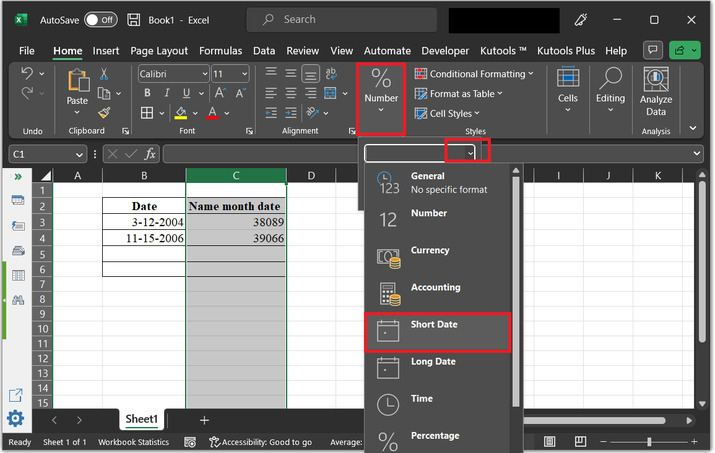### Step 6:

This will change the column format to short date, and the date will appear in proper format, as specified below: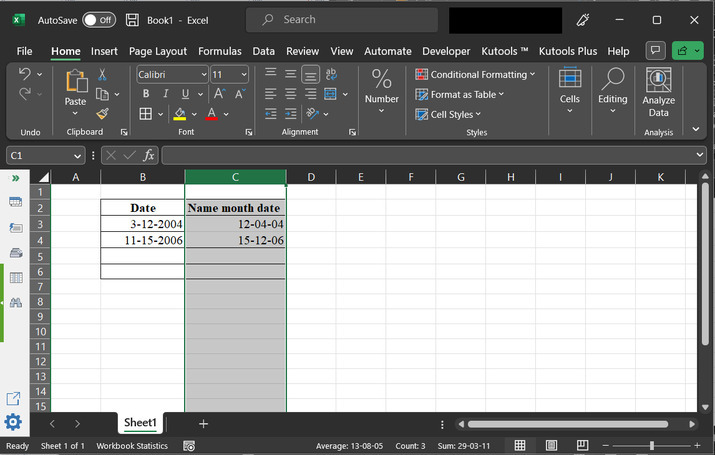### Step 7:

To change the date to the B column settings, again click on “Number” option, and select the “right corner arrow” as, highlighted below: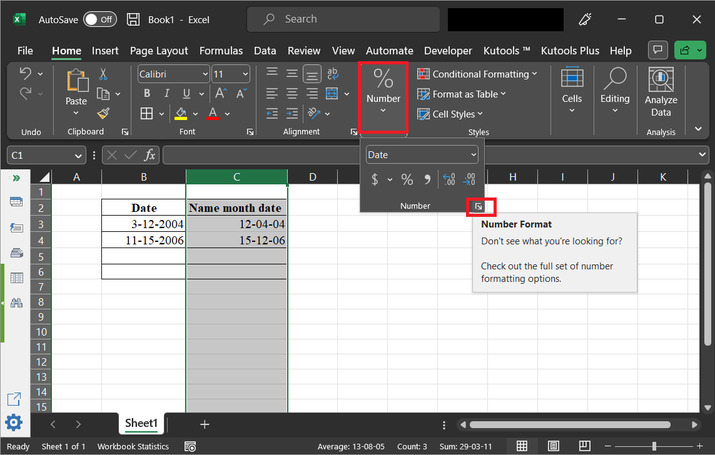### Step 8:

After that scroll down the options, and choose “3-14-2012”, and click on “Ok”. Consider below given image for reference: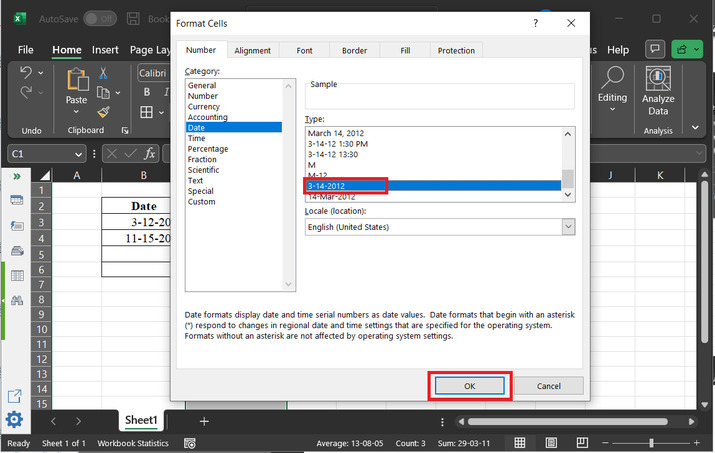### Step 9:

The finally, obtained output is depicted below: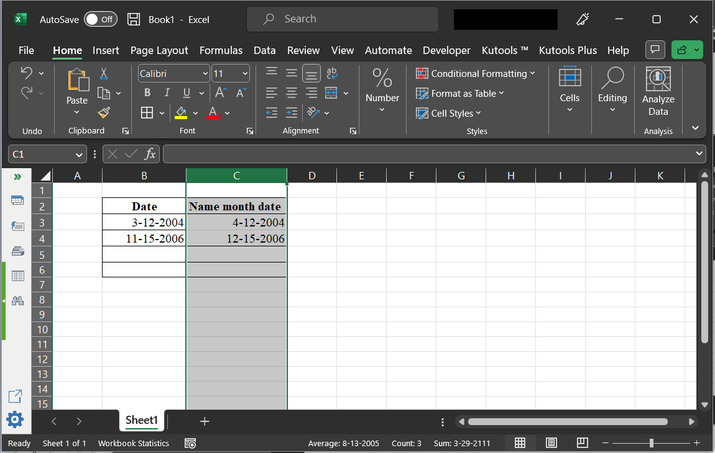## Example 2: To get the first day of the next month from the provided date in Excel by using the formula:

### Step 1:

Consider below-given spreadsheet, to understand all the steps precisely and accurately. Please note that the date format used in the below-given cells is “month-date-year”.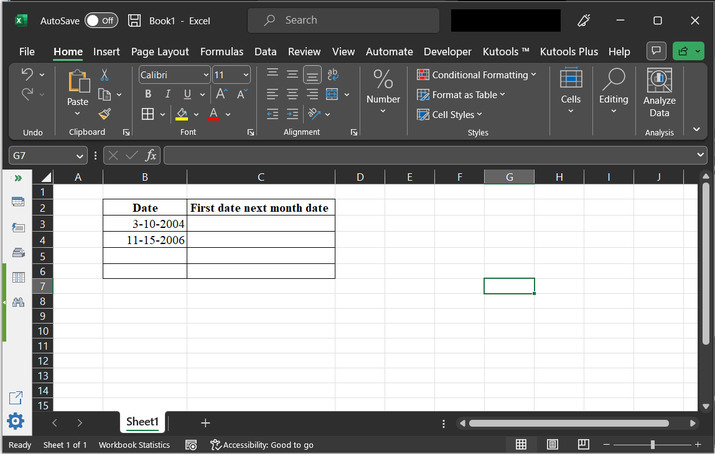### Step 2:

After that go to the column, where the learner is required to enter the next date of the month. For this example, the cell taken to generate output is C3.

In the cell type “=DATE(YEAR(B3),MONTH(B3)+1,1)”.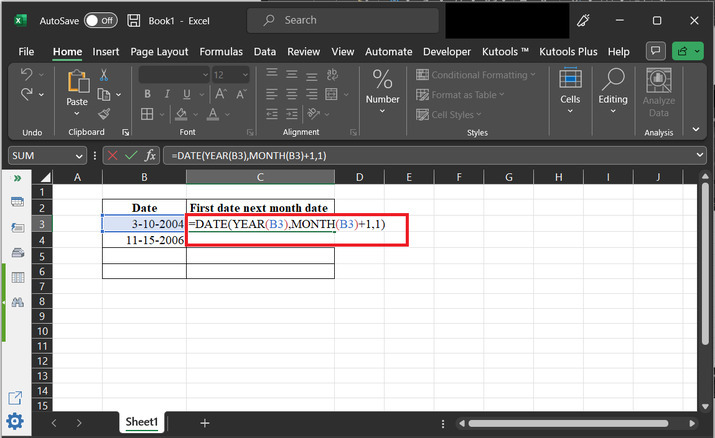### Explanation for formula:

=DATE(YEAR(B3),MONTH(B3)+1,1)

• YEAR(B3) This part will extract the year value from the date in cell B3.

• MONTH(B3)+1− this part of the code extracts the month value from the date in cell B3 and adds 1 to it. This is because the user needs to find the first day of the month that comes after the date in cell B3. By adding 1 to the month value. The evaluated value is part of next month.

• Finally, the number 1 is used as the day argument for the DATE Finally, the number 1 is used as the day argument for the DATE function. It will allow the learner to create a new date by combining the year, month, and day values.

Finally, the provided formula is used in Microsoft Excel to return a date that represents the first day of the month that comes after a specific date in cell B3.

### Step 3:

Finally press “Enter” key and then drag the cell to the C4 row, to copy same formula, to the next available row. The obtained results are provided below: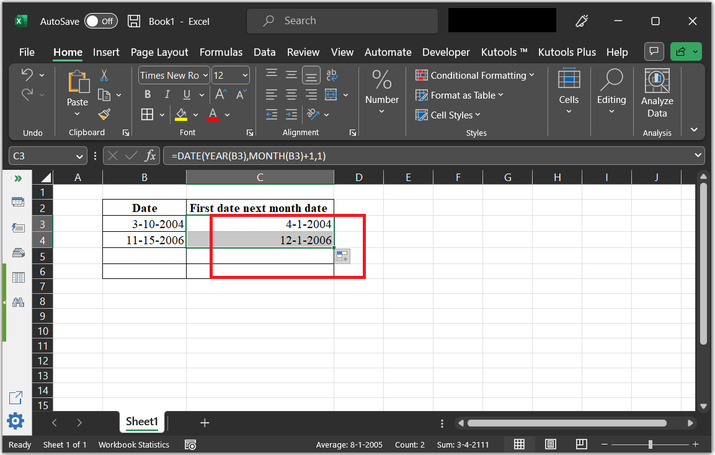## Conclusion:

By referring to the above two examples, the learner can easily understand the process of obtaining the next month's date, and the same day date of the next month. To perform both examples, the user can use the normal formula explained in the above context. Using the formula is considered one of the easiest practices to perform any task in Excel, as it is easy and can effectively generate accurate results.

Updated on: 17-Apr-2023

590 Views